## Introduction

Most evidence-based medical studies of causal relationship between an exposure and an outcome are based on observational data. However, the true causal effect between exposure and outcome is affected by confounders1, defined as variables that are associated with both exposure and outcome, but influenced by neither2. Without proper adjustment for confounders, the crude exposure-outcome association will be a biased estimate of the true association. Once identified, a confounder may be adjusted for by controlling it via appropriate study design techniques, such as restriction and matching of risk factors3, or by analyzing the exposure-outcome association with appropriate statistical techniques, such as regression4. The issue of confounding is not only limited to observational studies, as some researchers have even suggested that confounders should be adjusted for in analysis of randomized controlled trials data5,6. Therefore, confounder identification is an important issue in medical research.

Confounders can be identified by either empirical or theoretical strategies. Empirical strategies select a confounder based on objective criteria in the current working dataset. Examples of this strategy include forward, backward and stepwise regression4 and change-in-estimate (CIE) criterion7,8. With forward, backward and stepwise regression techniques, confounders are defined as those variables with a regression β on the outcome at a level of significance below a pre-specified. Popular choices for the pre-specified level of significance include 0.05, 0.10 and 0.201. With the CIE criterion, confounders are defined as those variables for which the percent difference between the values of the regression β when the variable is adjusted for compare with when it is not adjusted for is larger than a pre-specified value, usually 10%9. Use of the CIE criterion with a fixed cutoff level has been found inappropriate10 and some researchers recently proposed using a data-driven, simulated cutoff that yields a 5% of type I error rate10. Theoretical confounder identification strategies select the confounders from the results of previous studies or expert knowledge. This strategy is exemplified by directed acyclic graphs (DAGs)11.

In confounder identification, some researchers have relied on empirical criteria only when theoretical evidence is not available12, but other researchers have identified a need for combining empirical and theoretical criteria9,13. Simulation studies have shown that the CIE criterion combined with DAGs more accurately estimates exposure-outcome associations than do DAGs alone14. However, the decision of whether to adjust for remains unclear if the results from empirical and theoretical criteria are contradictory due to random error. These contradictions can be further divided into two cases, analogous to type I and type II errors. First, a variable without a confounding effect may show empirical confounding evidence in a particular dataset due to random error. Second, a true confounder may show no empirical confounding evidence. Most researchers have recommended first listing all theoretically possible confounders identified by DAGs and then selecting those that should be adjusted for by empirical methods13,14. However, these studies have provided no guidelines for handling confounders that are identified by empirical criteria but excluded in the DAGs; that is, potential confounders without theoretical background. How to handle these variables, and, equally, confounders suggested by DAGs that cannot be empirically validated, is a natural question. This study aims to answer the following research question “Should we adjust for a confounder if empirical and theoretical criteria yield contradictory results?” We simulated several scenarios in which a potential confounder exerted no confounding effect and other scenarios in which a true confounder exerted a confounding effect. Simulations were performed for different sample sizes and exposure-confounder correlations. By comparing the accuracy of exposure-outcome associations under different scenarios, we could determine when an adjustment for a potential confounder was required.

## Results

Table 1 summarizes the results of Simulation 1, in which a true confounding effect was present. In a simulation of size N = 200, the average probability of accurately detecting a confounding effect under the significance criterion (p < 0.05), significance criterion (p < 0.20), CIE criterion with a 10% cutoff and CIE criterion with a simulated cutoff was 9.77%, 29.32%, 12.32% and 59.14%, respectively. The probability decreased with increasing sample size under the CIE criterion with a 10% cutoff and increased with sample size under the significance criteria and the CIE criterion with a simulated cutoff. The significance criteria and the CIE criterion with a 10% cutoff underestimated the true OR, even when correctly identifying the confounding effect, although the performance of the significance criterion was better using the cutoff p-value of 0.20 compared with 0.05. Confounder adjustment led to underestimates as large as 16.63% (N = 500, CIE criterion with 10% cutoff, exposure-confounder association = 0.2), while no adjustment yielded overestimates as large as 6.64% (N = 200, CIE criterion with 10% cutoff, exposure-confounder association = 0.5). Under the significance criteria, however, these biases reduced as the sample size increased. While the significance criteria and the CIE criterion with a 10% cutoff performed poorly, the CIE criterion with a simulated cutoff provided accurate estimates. Under this criterion, the absolute percentage errors of the adjusted OR were all within 0.98% (the largest absolute percentage error was attained at N = 200, exposure-confounder association = 0.1). At a sample size of 1,000, all absolute percentage errors were within 0.2%. If no confounding effect was identified, the estimates were positively biased under all criteria. Under the significance criteria and the CIE criterion with a 10% cutoff, this bias was reduced by adjusting for the confounder, which reduced the percentage error by 4.28% at most (N = 1,000, CIE criterion with a 10% cutoff, exposure-confounder association = 0.5). To visualize the simulation results, the percentage error in the simulated OR for the case of N = 200 is shown in Figure 1.

Table 2 shows the results of Simulation 2, in which the potential confounder exerted no causal effect on the outcome. In a simulation of size N = 200, the average probability of accurately determining a null confounding effect under the significance criterion (p < 0.05), significance criterion (p < 0.20), CIE criterion with a 10% cutoff and CIE criterion with a simulated cutoff was 95.24%, 80.02%, 92.15% and 46.21%, respectively. This probability was independent of sample size under the significance criteria, decreased with increasing sample size under the CIE criterion with a 10% cutoff and increased with sample size under the CIE criterion with a simulated cutoff. Under all criteria, if no confounding effect was identified, the accuracy of the ORs was essentially unaltered by adjustment. The absolute differences in the percentage errors were all within 0.43% (the largest absolute difference in the percentage error was attained at N = 200, CIE criterion with 10% cutoff, exposure-confounder association = 0.5). However, if a confounding effect was identified, confounder adjustment increased the percentage error and RMSE. The percentage error in the simulated OR for the case of N = 200 is shown in Figure 2. Not surprisingly, the accuracy of the estimation improved with increasing sample size.

Table 3 shows the results of Simulations 3 and 4, in which the exposure and the potential confounder were uncorrelated. The average probability of accurately determining a null confounding effect was high under all four criteria (significance criterion (p < 0.05), significance criterion (p < 0.20), CIE criterion with a 10% cutoff and CIE criterion with a simulated cutoff). Provided that the potential confounder exerted no causal effect on the outcome (Simulation 3), these probabilities were independent of sample size; otherwise (Simulation 4), the probabilities reduced as sample size increased. As in the the results of Simulation 2, if no confounding effect was identified, the accuracy of the ORs was highly independent of imposed criterion and confounder adjustment. The percentage errors were all within 1.58% (the largest absolute percentage error was attained at N = 200, significance criterion, no confounder-outcome effect, confounder adjusted). Similarly, if a confounding effect was identified, no difference was found whether the confounder was adjusted for or not. Again, the accuracy of the estimation improved with increasing sample size.

Table 4 shows the results of Simulation 5, in which the potential confounder was affected by both the exposure and the outcome. The probability of correctly determining a null confounding effect was low under all criteria. Despite their poor performance in identifying a non-confounding effect, under all criteria the estimated OR for the exposure-outcome association were of acceptable accuracy. Even with a small sample size (N = 200), the absolute percentage error differences in the unadjusted ORs were all within 1.43% (the largest absolute difference was attained under all criteria, slope of regression line relating exposure to the potential confounder = 0.4). However, all adjusted ORs were positively biased and the biases increased with the slope of the regression line relating exposure to the potential confounder. The percent error in the simulated OR for the case of N = 200 is shown in Figure 3. As in Simulations 2 through 4, accuracy improved with increasing sample size.

## Discussion

Five different scenarios, based on various empirical and theoretical criteria, were simulated to evaluate the accuracy of exposure-outcome association estimates. Simulations containing a confounding effect and simulations without a confounding effect will be discussed in turn.

In the presence of a confounding effect, the OR estimates were biased under the significance criteria and the CIE criterion with a 10% cutoff. In simulations that indicated a confounding effect, the unadjusted OR was overestimated, but an adjustment for the confounding effect yielded underestimates of the true OR in subsequent logistic regression. These biases increased with increasing exposure-confounder correlation. The absolute percentage errors were largely unaffected by confounder adjustment. However, using the CIE criterion with a simulated cutoff yielded unbiased OR estimates. In simulations that indicated no confounding effect, adjustment only reduced part of the bias (the adjusted OR was less biased than the unadjusted OR). This result is expected under the significance criterion, which is subject to multi-collinearity effects. Under this criterion, adjusting for confounding is beneficial. However, we should note that the exposure-outcome association obtained from the dataset is biased regardless of confounder adjustment. The RMSEs yielded by all criteria were similar and were reduced with increasing sample size, as expected. Our simulation results agreed with previous simulation studies showing that a significance criterion using a p-value cutoff of 0.20 yielded better outcomes than that using a cutoff of 0.051.

To summarize the results of Simulation 1, theoretical confounders should be adjusted for regardless of the empirical evidence found in the dataset. This is consistent with previous simulation results, in which biases of estimates of exposure-outcome associations with uncontrolled confounders were reported15,16. Our simulation results showed that if a well-established confounding effect is not observed in a dataset, the results should be interpreted with caution, because the exposure-outcome association obtained from the dataset is likely to be biased.

In the absence of any confounding effect, all estimates were insensitive to the confounder identification criterion, to the adjustment for potential confounding and to the exposure-outcome association, although the biases increased with increasing exposure-confounder correlation. However, the RMSE increased when the confounding effect was identified and adjusted for. Under all criteria, the accuracy improved with increasing sample size. Under the significance criteria and the CIE criterion with a 10% cutoff, adjusting for the confounding effect induced marked increases in the RMSE. Under the significance criterion, the likelihood of adjustment was independent of sample size and exposure-confounder correlation, although these parameters affected the adjustment likelihood under the CIE criterion.

The results of Simulations 2, 3 and 4, in summary, suggested that the adjustment for factors that do not exert a confounding effect has a minimal impact on the accuracy of exposure-outcome estimates. Furthermore, all confounder identification criteria perform equally well in terms of percentage error and RMSE, except for the CIE with a 10% cutoff, which may yield a large percentage error in the estimation if a confounding effect is mistakenly identified. This minimal impact of adjusting for a factor without no confounding effect is unexpected; according to some researchers, variables that cause neither the exposure nor the outcome should not be adjusted for9,11, because empirical strategies for confounder identification cannot prove the existence of any casual effect between the confounder and the exposure or the outcome.

If a potential confounder is affected by both exposure and outcome, it is erroneously identified as a true confounder by all empirical identification criteria. This is expected because the potential confounder is associated with both exposure and outcome, which renders it indistinguishable from a true confounder if data-driven identification strategies are used. Therefore, to summarize the results of Simulation 5, we should not adjust for a potential confounder that is affected by both exposure and outcome. Instead, we should implement a priori confounder selection by DAGs9,11.

The simulation results showed us that adjusting for a non-confounder has a minimally adverse impact in the estimation of the exposure-outcome association. Therefore, we should adjust for a potential confounder even if there is little or no theoretical background knowledge of its confounding effect. However, cautions must be taken in adjusting for variables that are associated with both exposure and outcome because adjusting for such variables will lead to a biased estimation of the exposure-outcome association.

Previous simulation studies have shown that while both significance criteria and CIE criteria can be implemented for confounder identification, CIE criteria works better than significance criteria8,17. Similar conclusions can be drawn in this study. Comparing the percentage errors and RMSEs across different simulations shows that the CIE criterion with a simulated cutoff clearly outperformed the other three criteria in detecting a confounding effect while yielding the highest accuracy in estimating the true association between exposure and outcome. The CIE criterion with a fixed cutoff of 10% showed diminishing power for determining confounding effects as the sample size increased; at a sample size of 1,000 and an exposure-confounder correlation of <0.2, the power dropped to almost zero. However, the CIE criterion with a simulated cutoff achieved a power of 80% in most of the scenarios. The cutoff point of 10% appeared to be too high, as the simulations yielded cutoff points of 0.4% to 2.5% for an OR of 1.1 and cutoff points of 0.9% to 5.4% for an OR of 1.6.

This study was not without limitations. One such limitation was that we implemented adjustment by including only a linear term in the logistic regression. Other forms of confounding, for instance confounding by a categorical variable, could have different effects and such effects should be simulated in future studies. Because adjustment for the confounder with a linear effect model yielded satisfactory estimates of the true exposure-outcome association in prior simulation studies18, we did not consider other forms of confounding here. A second limitation arises from the fact that evidence-based medical studies often involve ordinal, continuous and survival outcomes. Although only the stimulation results of binary outcomes were studied here, further simulation studies using other types of outcomes and different exposure-outcome effects are warranted, because the confounder adjustment properties differ among regression models10,19. Additionally, the conclusions in this study were drawn based on a limited range of parameters and it remains unknown what the results will be for parameters out of the range this study tested. Such simulations could be performed by modifying the R code provided in Additional file 1. A third limitation is that several less common empirical confounder identification strategies, including Akaike information criterion and Bayesian information criterion1, have not been tested in this simulation study. Again, interested readers could test these strategies with a slight modification of the provided R code. A final limitation is that the causal effect of an outcome, especially a health-related outcome, often involves the interaction of several causal exposures and is seldom due to a single exposure20. The scenarios simulated in this study were simplifications of real situations and the conclusions should not be generalized to multiple-exposure effects.

## Methods

Here, we denote exposure, outcome and the possible confounder by X, Y and Z, respectively. Z is a confounder if it satisfies the following conditions2: (a) X is associated with Z, (b) Y is associated with Z and (c) Z is not caused by both X and Y.

In what follows, we will assume the effects of Z and X on Y are linear and compare the estimated effect of X on Y using linear regression with and without adjusting for Z. Note that the relationships between X, Y and Z can be stated mathematically as Y = βX,YX + βY,ZZ + ε, where ε is the error term. If a linear regression is fitted on Y with only X as the independent variable, that is, Y = βX,Y’X + ε’, then the maximum likelihood estimator (MLE) of βX,Y’, βX,Y’*, equals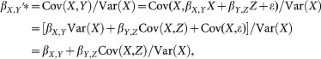assuming that X and ε are uncorrelated. Hence, βX,Y’* is an unbiased estimator of βX,Y if and only if Cov(X, Z) = 0 or βY,Z = 0 (making the second term in the right hand side of the equation zero). Thus, βX,Y’* is an unbiased estimator of βX,Y if and only if either condition (a) or (b) is not satisfied, or in other words, Z is not a confounder of the association between X and Y. This result shows that a true confounder should be adjusted for, otherwise the estimation of exposure-outcome association will be biased. This result also shows that adjusting for a variable with no association with either the exposure or the outcome has no effect on the bias of the exposure-outcome association estimate. Of course, the standard error of βX,Y’* may be higher than that of βX,Y*, which is the MLE of βX,Y, depending on the correlation between X and Z. It is clear that a potential confounder should not be adjusted for based only on its association with the outcome because if Cov(X,Z) = 0 the unadjusted MLE βX,Y’* is an unbiased estimator of βX,Y.

The CIE criterion with a 10% cutoff to determine whether a potential confounder should be adjusted for can be restated as βY,ZCov(X, Z)/βX,YVar(X) > 10%. If there exists a confounding effect but the estimate of βY,ZCov(X, Z)/βX,YVar(X) is less than 10%, the bias could be due to the underestimation of βY,Zand/or Cov(X, Z) or to the overestimation of Var(X) and we cannot determine which. Hence, we cannot determine which estimator, βX,Y’* or βX,Y*, is a better estimate of βX,Y; therefore we use simulations to answer this question.

Furthermore, the aforementioned theory only applies to linear regressions. However, for logistic regression and other types of regression in which that the MLEs have no closed-form solution, the biased of the MLEs due to an unadjusted confounding effect could not be determined using the above proof, therefore we try to answer the above questions using simulations.

### Disagreement of empirical and theoretical confounder adjustment criteria due to random error

This study aims to investigate whether to adjust for a potential confounder or not when empirical and theoretical confounder adjustment criteria disagree due to random error. This is not uncommon, as is illustrated with the following simulation. A total of 10,000 datasets were simulated with sample sizes of N = 500. In these datasets, a random variable Z, which followed a normal distribution with a mean of zero and a variance of one, was generated and Y was generated according to the equation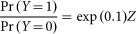. The p-values of the fitted odds ratios (OR)s are plotted as a histogram in Figure 4. It can be deduced that the power of the logistic regression was small. Even under the least stringent significance criterion of p < 0.20, the association between Z and Y was identified empirically in less than 50% of the simulated datasets. While it is obvious that we should adjust for Z (according to the significance criterion and/or prior knowledge that Z and Y are correlated) within those datasets where Z and Y were associated, the decision to adjust for Z in those datasets where Z and Y were not associated, is yet to be determined. Previous studies on confounder adjustment did not consider such a problem and this study aims to provide guidelines for this situation and for other similar situations where empirical and theoretical confounder adjustment criteria yield conflicting results due to random error.

### Model simulation

In the following simulations, we assumed no selection bias, that all errors were random and that there were no systematic errors such as measurement errors. We simulated the case for logistic regression and the accuracy of the estimated OR for the effect of X on Y with and without confounder adjustment was compared in five simulations with different correlation patterns between X and Z and between Y and Z imposing different objective criteria. In the first four simulations, X and Z were both normally distributed with zero mean and variance of one. X and Z were drawn to satisfy specific Pearson correlations. In Simulation 1, Y was a binary variable generated according to the equation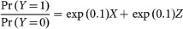. In Simulation 2, Y was generated according to the equation. In both simulations, X and Z were correlated to varying extents (0.1, 0.2, 0.3, 0.4 and 0.5). The data for Simulations 3 and 4 were generated similarly but with no correlation between X and Z. In Simulation 5, X was normally distributed with a mean of zero and a variance of one, Y was generated according to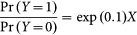and Z was specified by βX + Y + ε, where ε is a random variable drawn from a normal distribution with a mean and variance of zero and one, respectively. Different levels of β (0.1, 0.2, 0.3, 0.4 and 0.5) were simulated. We noted that Z was a true confounder in Simulation 1, while Simulations 2, 3 and 4 mimicked a logistic regression in which Z was not a confounder. Z was affected by both X and Y in Simulation 5. Simulations were conducted at different sample sizes (200, 500 and 1,000) and 10,000 datasets were simulated in each scenario.

The OR of the above simulations were set to exp(0.1) ~ 1.1. Additionally, both the exposure and the confounder were continuous. An additional set of simulations were performed with ORs = exp(0.5) ~ 1.6 and both the exposure and the confounder were dichotomized with positive values and negative values set to 1 and 0, respectively. The conclusions drawn from the two sets of simulations were similar, therefore only the first set of simulations with ORs = exp(0.1) were reported here. The results of the additional set of simulations were provided in the Supplementary Information.

### Estimation of the OR

Under all five scenarios, the 10,000 simulated datasets were divided into two groups. The first group was composed of those simulated datasets that showed empirical evidence of a need for confounder adjustment. This evidence was based on significance (a potential confounder was adjusted for if the p value of its OR was below 0.05 or 0.20), on the CIE criterion with a 10% cutoff (a potential confounder was adjusted for if the adjustment changed the OR by more than 10% of the unadjusted OR), or on the CIE criterion with a simulated cutoff10 yielding a 5% level of type I error (that is, a variable without confounding effect has a probability of 5% to be identified as a confounder). Each of these adjustments was performed separately. Those datasets that showed no empirical evidence of a need for confounder adjustment belonged to the second group. We reported the percentage of the simulated datasets showing evidence of confounder adjustment. Two regression models were fitted to both groups. Both models treated Y as the dependent variable (denoted by ORx and ORx’ for datasets in the first and second group respectively). However, one model assumed that X was the sole independent variable, while the other further adjusted for Z (denoted by ORx,z and ORx,z’ for datasets in the first and second group, respectively).

### Performance assessment

The estimation accuracy was assessed from the percentage error, given by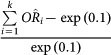and the root-mean-square error (RMSE), given by, where k and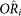are the total number of simulations and the estimated OR for the ith simulation respectively. All simulations were carried out using R version 3.0.0 and the simulation syntax is given in the Supplementary Information.